Select Page

In a previous couple of articles, we explored some basic machine learning algorithms. Thus far we covered some simple regression algorithms, classification algorithms and we started with algorithms that can be used for both types of problems. We used technologies like TensorFlow, Pytorch and SciKit Learn for the implementation and application of these algorithms. Apart from that we used optimization techniques such as Gradient Descent. So far we have covered:

In this article, we explore Decision Trees. Just like SVM, Decision Tree is capable of performing both classification and regression tasks. They are one of the most popular machine learning algorithms. The secret of its popularity lies within its simplicity. Also, they are able to produce results with a small amount of data and solutions it provides are easily explainable. This algorithm is exceptionally useful when it is used in ensemble learning, ie. they are an integral part of Random Forest, one of the most powerful machine learning algorithms. Random Forest is just a set of multiple Decision Trees, but more on that later.

Are you afraid that AI might take your job? Make sure you are the one who is building it.

STAY RELEVANT IN THE RISING AI INDUSTRY! 🖖

## Dataset and Prerequisites

Data that we use in this article is from PalmerPenguins Dataset. This dataset has been recently introduced as an alternative to the famous Iris dataset. It is created by Dr. Kristen Gorman and the Palmer Station, Antarctica LTER. You can obtain this dataset here, or via Kaggle. This dataset is essentially composed of two datasets, each containing data of 344 penguins. Just like in Iris dataset there are 3 different species of penguins coming from 3 islands in the Palmer Archipelago. Also, these datasets contain culmen dimensions for each species. The culmen is the upper ridge of a bird’s bill. In the simplified penguin’s data, culmen length and depth are renamed as variables culmen_length_mm and culmen_depth_mm.Data that we use for the regression examples we use the famous Boston Housing Dataset. This dataset is composed 14 features and contains information collected by the U.S Census Service concerning housing in the area of Boston Mass. It is a small dataset with only 506 samples.

For the purpose of this article, make sure that you have installed the following Python libraries:

• NumPy – Follow this guide if you need help with installation.
• SciKit Learn – Follow this guide if you need help with installation.

Once installed make sure that you have imported all the necessary modules that are used in this tutorial.

``````import pandas as pd
import numpy as np
import matplotlib.pyplot as plt

from sklearn.model_selection import train_test_split
from sklearn.metrics import accuracy_score
from sklearn.tree import DecisionTreeClassifier, DecisionTreeRegressor, export_graphviz
from sklearn.ensemble import RandomForestClassifier, RandomForestRegressor``````

Apart from that, it would be good to be at least familiar with the basics of linear algebra, calculus and probability.

## Decision Trees for Classification and Regression

In essence, Decision Tree is a set of algorithms, because there are multiple ways in which we can solve this problem. Some of the most famous ones are:

• CART
• ID3
• C4.5
• C5.0

In this article, we focus on the CART algorithm which is easies and one of the most popular ones. Among others, the Sci-Kit Learn library uses this algorithm under the hood. This algorithm produces a binary tree, which might not be the case with other algorithms. This means that the node is either branching in two nodes or it is not branching at all (terminal node or terminal leaf). Here is the preview of how CART builds a Decision Tree.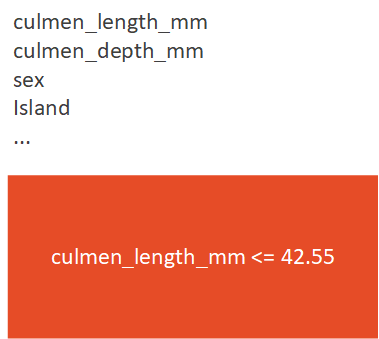In the beginning, it adds the root node of the three and we push all data to it. In this first node, we examine the value of one of the features. In the example of PalmerPenguins let’s say it examines culmen_length_mm feature and compares it with the chosen threshold, in this case, 42.55. Thus, data is partitioned into two sets. The first one for which this question is true, and the other one for which it is not. Then two new nodes are created which are examining some other feature and uses some other thresholds:The process is then repeated. The depth of the tree is controlled by the max_depth hyperparameter. How the thresholds are created? To understand that we need to explain two important concepts: impurity and information gain. Impurity can be defined as a chance of being incorrect if you assign a label to an example by random. This means that a node is “pure” if all training instances it applies to belong to the same class, meaning when you assign a label to a random sample you can not make a mistake. There are different ways for measuring impurity such a Gini index and entropy. In this article, we use the Gini index. To calculate Gini impurity index we use the formula:where pi,k is the ratio of class k instances among the training instances in the i-th node. For example, if we have 43 instances of the training set in the node of which 13 belong to one class, while 30 instances belong to a second class. Given that we have only those two classes in the training dataset, we calculate Gini impurity 1 – (13/43)2 – (30/43)2 ≈ 1 – 0.09 – 0.49 ≈ 0.42. When the node is “pure” its Gini index is 0.

On the other hand, information gain lets us find the best threshold which will reduce this impurity the most. To calculate information gain we need to calculate average impurity and then subtract that from the starting impurity. That is how we know the quality of thresholds that we used.

Based on these two concepts we can define how the CART algorithm functions. In its essence, it is a greedy algorithm that repeats the process for each level (depth). First, it splits the training dataset into two subsets using single feature j and a threshold tj. The feature and the threshold are picked like that so that they produce the purest subsets weighted by their size. The cost function that CART minimizes can be defined as: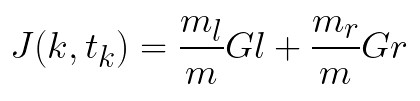where ml and mr represent the number of instances in the respective side (right, left), m is the total number of instances and Gl and Gr represent the Gini impurity index on the respective side. Once this is done, it does the same to each subset. The process is repeated recursively until the maximum depth is reached or a split that reduces impurity can not be found. This algorithm can be used for both regressions and for classification. The only difference is that in one case the resulting decision is the class of the sample, while in the other is the value of the sample. Also, instead of trying to reduce the Gini impurity index, for regression tasks MSE (mean squared error) is used: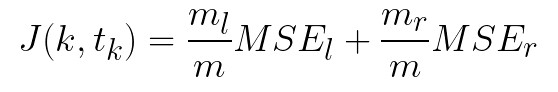### Decision Tree Classifier Python Implementation From Scratch

As we mentioned for the classification examples we use PalmerPenguins dataset. However, since we want to do binary classification we need to do some preparations. First, we load the dataset, remove features that we don’t use in this article and remove the one class because we perform binary classification:

``````data = pd.read_csv('./data/penguins_size.csv')We remove all samples that are labeled with class ‘Chinstrap‘ and features that we don’t want to use (we use only culmen_length_mm and culmen_depth_mm).

``````data = data.dropna()
data = data.drop(['sex', 'island', 'flipper_length_mm', 'body_mass_g'], axis=1)
data = data[data['species'] != 'Chinstrap']``````

Then we separate input data and scale it:

``````X = data.drop(['species'], axis=1)
X = X.values
ss = StandardScaler()
X = ss.fit_transform(X)``````

After that, we extract output values and mark them with values -1 and 1, since the SVM algorithm requires that.

``````y = data['species']
spicies = {'Adelie': -1, 'Gentoo': 1}
y = [spicies[item] for item in y]
y = np.array(y) ``````

Another thing that we remove is the 182nd sample form the dataset. Why? Well, this sample was in between classes and it kinda messed with points that we try to make, so we remove it and split data into training and test datasets:

``````# Remove sample that is too close
X = np.delete(X, 182, axis=0)
y = np.delete(y, 182, axis=0)

X_train, X_test, y_train, y_test = train_test_split(X, y, test_size=0.2, random_state=33)``````

That is it, our dataset is ready and here is what it looks when we plot it:

``````plt.figure(figsize=(11, 5))
plt.scatter(X[y == 0][:, 0], X[y == 0][:, 1], color='orange', label='Adelie')
plt.scatter(X[y == 1][:, 0], X[y == 1][:, 1], color='gray', label='Gentoo')
plt.legend();``````Ok, once that is done, we can implement helper class MyGiniCalculator. This class is used for the Gini impurity index calculations we mentioned in the previous chapter:

``````class MyGiniCalculator():

def _single_class_gini(self, class_count, total_instances_count):
return -(class_count/total_instances_count)

def _group_gini(self, class1_count, class2_count):
if class1_count == 0 or class2_count == 0:
return 0

total_count = class1_count + class2_count
return self._single_class_gini(class1_count, total_count) + self._single_class_gini(class2_count, total_count)

def _leaf_gini(self, data):
gini = 0

num_istances = len(data)
classes = set(data)

for classs in classes:
class_count = sum(data == classs)
gini += class_count * 1.0/num_istances * self._group_gini(sum(data == classs), sum(data != classs))

return gini, num_istances

def get_gini(self, is_left, y):
if len(is_left) != len(y):
print('Wrong length')
return None

num_istances = len(y)
left_gini, left_count = self._leaf_gini(y[is_left])
right_gini, right_count = self._leaf_gini(y[~is_left])

total_gini = left_count*1.0/num_istances * left_gini + right_count*1.0/num_istances * right_gini

Note that this class has several methods, of which only one is public:

• _single_class_gini – Calculates the Gini impurity index for a single class
• _group_gini – Since we build a binary tree this method calculates the Gini impurity index for a group of two classes
• _leaf_gini – This method calculates the Gini impurity index for one leaf
• get_gini – This method calculates the Gini impurity index

Awesome, this class helps us a lot when it comes to implementation of the MyDecissionTreeClassifier class, which contains the implementation of Decision Tree:

``````class MyDecissionTreeClassifier():
def __init__(self, feature_names, max_depth = 5):
self.gini_calculator = MyGiniCalculator()
self.feature_names = feature_names
self.max_depth = max_depth
self.depth = 0

def _get_best_feature_split(self, feature, y):
min_gini = 10

for value in set(feature):
is_left = feature < value
gini = self.gini_calculator.get_gini(is_left, y)
if gini < min_gini:
min_gini = gini
cutoff = value

return min_gini, cutoff

def _get_best_split(self, X, y):
feature = None
min_gini = 1
cutoff = None

for i, c in enumerate(X.T):
gini, cur_cutoff = self._get_best_feature_split(c, y)

if gini == 0:
return i, cur_cutoff, gini
elif gini <= min_gini:
min_gini = gini
feature = i
cutoff = cur_cutoff

return feature, cutoff, min_gini

def _get_prediction(self, row):
cur_layer = self.trees

while cur_layer:
prev_layer = cur_layer

if row[cur_layer['index']] < cur_layer['cutoff']:
cur_layer = cur_layer['left']
else:
cur_layer = cur_layer['right']
else:
return prev_layer.get('value')

def fit(self, X, y, parent_leaf={}, depth=0):

if parent_leaf is None or len(y) == 0 or depth >= self.max_depth:
return None

feature, cutoff, gini = self._get_best_split(X, y)
y_left = y[X[:, feature] < cutoff]
y_right = y[X[:, feature] >= cutoff]

parent_leaf = {
'feature': self.feature_names[feature],
'index': feature,
'cutoff': cutoff,
'value': np.round(np.mean(y))}

parent_leaf['left'] = self.fit(X[X[:, feature] < cutoff], y_left, {}, depth+1)
parent_leaf['right'] = self.fit(X[X[:, feature] >= cutoff], y_right, {}, depth+1)

self.depth += 1
self.trees = parent_leaf

return parent_leaf

def predict(self, X):
results = np.array(*len(X))

for i, row in enumerate(X):
results[i] = self._get_prediction(row)

return results``````

Ok, there are a lot of bits and pieces that need to be explained here. Let’s split this class into smaller chunks and start form the constructor:

``````def __init__(self, feature_names, max_depth = 5):
self.gini_calculator = MyGiniCalculator()
self.feature_names = feature_names
self.max_depth = max_depth
self.depth = 0``````

Here we initialize several fields, of which max_depth is the most important one. It is the hyperparameter that controls the ‘greediness‘ of our algorithm. Of course, we create instances of MyGiniCalculator. Next method is _get_best_feature_split:

``````def _get_best_feature_split(self, feature, y):
min_gini = 10

for value in set(feature):
is_left = feature < value
gini = self.gini_calculator.get_gini(is_left, y)
if gini < min_gini:
min_gini = gini
cutoff = value

return min_gini, cutoff``````

This a private method, used to get the split of the feature. Basically based on the value of the Gini impurity index for that feature, we calculate the threshold or cutoff value. This method is used in _get_best_split:

``````def _get_best_split(self, X, y):
feature = None
min_gini = 1
cutoff = None

for i, c in enumerate(X.T):
gini, cur_cutoff = self._get_best_feature_split(c, y)

if gini == 0:
return i, cur_cutoff, gini
elif gini <= min_gini:
min_gini = gini
feature = i
cutoff = cur_cutoff

return feature, cutoff, min_gini``````

This private method is used to iterate through all features, calculate the best thresholds and features on which the split should be done on each depth of the tree. Basically, this method not only calculates the Gini impurity indexes for all features but picks the one feature that minimizes the Gini impurity index the most. The final private method is _get_prediction:

``````def _get_prediction(self, row):
cur_layer = self.trees

while cur_layer:
prev_layer = cur_layer

if row[cur_layer['index']] < cur_layer['cutoff']:
cur_layer = cur_layer['left']
else:
cur_layer = cur_layer['right']
else:
return prev_layer.get('value')``````

This method is once predictions are made. The two public methods are very interesting. Let’s start with the fit method:

``````def fit(self, X, y, parent_leaf={}, depth=0):

if parent_leaf is None or len(y) == 0 or depth >= self.max_depth:
return None

feature, cutoff, gini = self._get_best_split(X, y)
y_left = y[X[:, feature] < cutoff]
y_right = y[X[:, feature] >= cutoff]

parent_leaf = {
'feature': self.feature_names[feature],
'index': feature,
'cutoff': cutoff,
'value': np.round(np.mean(y))}

parent_leaf['left'] = self.fit(X[X[:, feature] < cutoff], y_left, {}, depth+1)
parent_leaf['right'] = self.fit(X[X[:, feature] >= cutoff], y_right, {}, depth+1)

self.depth += 1
self.trees = parent_leaf

return parent_leaf ``````

This method performs the training process. Once input and output values are passed into this method, it gets the best split and stores it into the parent_leaf dictionary. Every leaf is essentially abstracted by one dictionary and every leaf has its left and right leaf (except for terminal ones). So, for the first level, we calculate the parent leaf split. Then based on the feature and values on this level, we recursively create left and right leaf based on these values. This is how we build a tree. Once training is done, we can use the predict method:

``````def predict(self, X):
results = np.array(*len(X))

for i, row in enumerate(X):
results[i] = self._get_prediction(row)

return results``````

This method is fairly simple. It utilizes _get_prediction, which follows the path to the result. Here is how we can call this class on the prepared dataset:

``````model = MyDecissionTreeClassifier(feature_names=data.drop(['species'], axis=1).columns)
tree = model.fit(X_train, y_train)
preditions = model.predict(X_test)
print(accuracy_score(preditions, y_test))``````
``0.9433962264150944``

The accuracy_score is ~0.94, which is not that bad.

### Using SciKit Learn

Of course, the faster way is to use the Sci-Kit Learn library for this purpose and their DecisionTreeClassifier:

``````dt_model = DecisionTreeClassifier(max_depth=2)

dt_model.fit(X_train, y_train)

dt_preditions = dt_model.predict(X_test)
print(accuracy_score(dt_preditions, y_test))``````
``1.0``

With this class we get 100% accuracy. If we compare the results:

``````pd.DataFrame({
'Actual Value': y_test,
'My Decision Tree Predictions': preditions,
'DecisionTreeClassifier Predictions': dt_preditions
})``````One more cool thing we can do with Sci-Kit Learn’s Decision Trees is that we can export them into .dot file using export_graphviz:

``````export_graphviz(
dt_model,
out_file="penguins.dot",
feature_names=data.drop(['species'], axis=1).columns,
class_names='species',
rounded=False,
filled=True
)``````

This file can be opened here. Here is the result: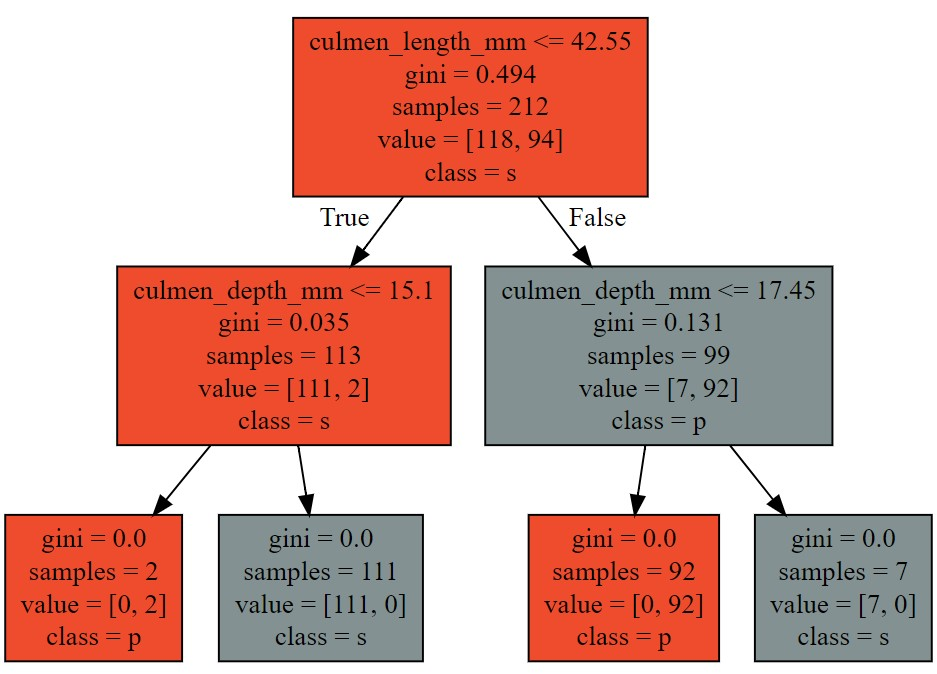It is a very cool visual representation of the created Decision Tree and it leafs. In this diagram, we can see every leaf Gini index, feature with threshold used and the number of samples. Another cool thing we can do is present the split on the data diagram: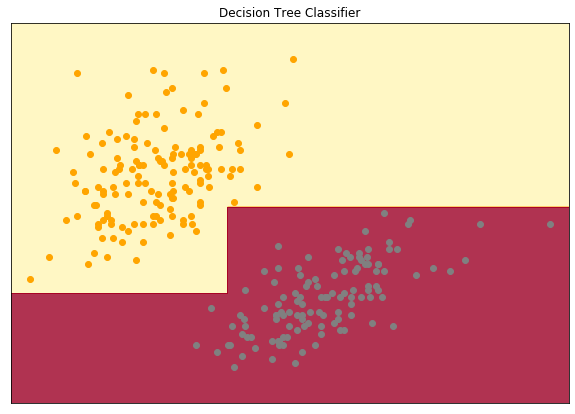We can see how Decision Trees make almost perfect splits on the data. This is probably one of the major downfalls of these algorithms, they are prone to overfitting.

## Decission Tree Regression

The really cool thing about Decision Trees is that they can be used for regression too. Let’s load the Boston Housing dataset and check out how that is done:

``````data = pd.read_csv('./data/boston_housing.csv')

data = data.dropna()

X = data['lstat'].values
X = X.reshape(-1, 1)

y = data['medv'].values

X_train, X_test, y_train, y_test = train_test_split(X, y, test_size=0.2, random_state=33)

plt.scatter(x = X, y = y, color='orange')
plt.show()``````Data is fairly simple. This dataset contains 14 features, of which we use 2. Our goal in this example is to predict the value of the property based on the age of the occupant. So, we remove all other features. As you can see this is the case of non-linear regression. So, let’s try to create a model that will best fit this data.

### Using Sci-Kit Learn

Once again, the Sci-Kit Learn helps us do this:

``````dtr_model = DecisionTreeRegressor(max_depth=5)
dtr_model.fit(X_train, y_train)

dtr_predictions = dtr_model.predict(X_test)

pd.DataFrame({
'Actual Value': y_test,
'DecisionTreeRegressor Prediction': dtr_predictions,
})``````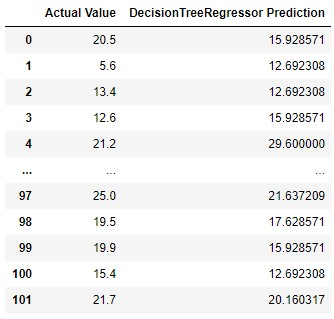We can see that results are not bad and it is really interesting once we plot it:## Random Forest and Ensamble Learning

The interesting occurrence in machine learning is that sometimes we tend to get better results by using multiple predictors and then averaging results than from using one special algorithm for it. This technique in which we use multiple algorithms instead of one is called Ensemble Learning. Ensemble Learning is based on the law of the large numbers, which means that even if algorithms that are composing the ensemble are weak learners, the ensemble can be a strong learner. There are several ways these ensemble learners function. For example, in the technique called hard voting, several classifiers vote for the class and the class that gets the majority of the votes is the output. This is a bit unintuitive, but if you build an ensemble containing 1,000 classifiers and each of them has an accuracy of 51% on its own, assemble based on hard-voting can have accuracy up to 75%. There is also a soft voting technique. In this case, each algorithm outputs probability, the ensemble will predict the class with the highest class probability, averaged over all the individual classifiers.One of the most popular ways to build ensembles is to use the same algorithm multiple times but on the different subsets of the training dataset. Techniques that are used for this are called bagging and pasting. The only difference in these techniques is that while building subsets bagging allows training instances to be
sampled several times for the same predictor, while pasting is not allowing that. When all algorithms are trained, the ensemble makes a prediction by aggregating the predictions of all algorithms. In the classification case that is usually the hard-voting process, while for the regression average result is taken.

Random Forest is one of the most powerful algorithms in machine learning. It is an example of Decision Trees. In most of the cases, we train Random Forest with bagging to get the best results. It introduces additional randomness when building trees as well, which leads to greater tree diversity. This is done by the procedure called feature bagging. This means that for each tree during the training is trained on a different subset of features. In turn, this leads to lower variance of the complete model. This should be enough of the theory, let’s implement RandomForest from scratch using Python and Decision Tree we implemented in the previous chapter.

### Classification Python Implementation From Scatch

The complete algorithm is implemented within MyRandomForest class:

``````class MyRandomForest():
def __init__(self, num_trees, feature_names, max_depth=5):
self.num_trees = num_trees
self.max_depth = max_depth

self.trees = []
for _ in range(num_trees):
self.trees.append(MyDecissionTreeClassifier(feature_names, self.max_depth))

def get_random_subsets(self, X, y, num_subsets):
num_samples = np.shape(X)

X_y = np.concatenate((X, y.reshape((1, len(y))).T), axis=1)
np.random.shuffle(X_y)

subsets = []
subsample_size = int(num_samples // 2)

for _ in range(num_subsets):
index = np.random.choice(
range(num_samples),
size=np.shape(range(subsample_size)),
replace=True)

X = X_y[index][:, :-1]
y = X_y[index][:, -1]

subsets.append([X, y])

return subsets

def fit(self, X, y):
num_features = np.shape(X)
subsets = self.get_random_subsets(X, y, self.num_trees)

for i in range(self.num_trees):
X_subset, y_subset = subsets[i]

# Feature bagging
idx = np.random.choice(range(num_features), size=num_features, replace=True)
self.trees[i].feature_indices = idx
X_subset = X_subset[:, idx]
self.trees[i].fit(X_subset, y_subset)

def predict(self, X):
y_preds = np.empty((X.shape, len(self.trees)))

for i, tree in enumerate(self.trees):
idx = tree.feature_indices
prediction = tree.predict(X[:, idx])
y_preds[:, i] = prediction

y_pred = []
for sample_predictions in y_preds:
y_pred.append(np.bincount(sample_predictions.astype('int')).argmax())

return y_pred``````

In the constructor of the class, we create an array of MyDecissionTreeClassifier instances and define max_depth for each of them. Apart from that, we set the max_features hyperparameter used for feature bagging. In the get_random_subsets method, we create a defined number of subsets, which are later used to train each individual tree. The fit method is fairly simple. For each tree, we create a subset of data, perform feature bagging and run the training process. Let’s see what we get when we use this on PalmerPenguins dataset:

``````model = MyRandomForest(feature_names=data.drop(['species'], axis=1).columns, \
num_trees=11, max_features=2)
tree = model.fit(X_train, y_train)

preditions = model.predict(X_test)
print(accuracy_score(preditions, y_test))``````
``0.9622641509433962``

As you can see we get accuracy improvement from using just MyDecissionTreeClassifier.

### Classification with Sci-Kit Learn

Sci-Kit Learn library provides a better implementation of this algorithm. Here is how we can use RandomForestClassifier:

``````rf_classifier = RandomForestClassifier(n_estimators=11, max_leaf_nodes=16, n_jobs=-1)
rf_classifier.fit(X_train, y_train)

rf_preditions = rf_classifier.predict(X_test)
print(accuracy_score(rf_preditions, y_test))``````
``1.0``

Let’s check out how the classification diagram for Random Forest looks like and what are the differences from the Decision Trees one:Ok, that looks much better. It seems that Random Forest got a better feel of where is the border between classes.

### Regression with Sci-Kit Learn

Classification seems simple enough, but just like Decision Trees, Random Forest can be used for regression too. Again, bagging is used during training, but instead of the voting process, the results of all learners are averaged. Here is how we can use it for the Boston Housing dataset:

``````rf_classifier = RandomForestClassifier(n_estimators=11, max_leaf_nodes=16, n_jobs=-1)
rf_classifier.fit(X_train, y_train)

rf_preditions = rf_classifier.predict(X_test)
print(accuracy_score(rf_preditions, y_test))``````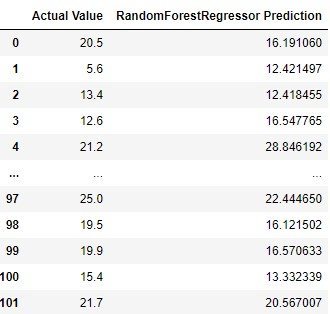Again, compared to Decision Tree results it seems a bit better. Graphically it looks better too: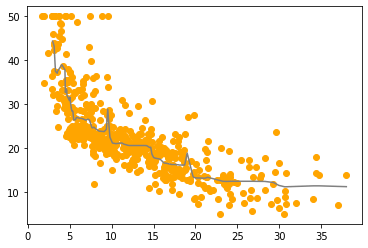## Conclusion

In this article, we covered a lot of ground. We learned how Decision Trees work and how are they built with CART algorithm with Gini impurity index. Also, we had a chance to see how Ensamble Learning functions and how we can build Random Forest from Decission Tree algorithms. As always, we implemented all from scratch in Python and used Sci-Kit Learn for simplification.#### Nikola M. Zivkovic

CAIO at Rubik's Code

Nikola M. Zivkovic a CAIO at Rubik’s Code and the author of book “Deep Learning for Programmers“. He is loves knowledge sharing, and he is experienced speaker. You can find him speaking at meetups, conferences and as a guest lecturer at the University of Novi Sad.

Rubik’s Code is a boutique data science and software service company with more than 10 years of experience in Machine Learning, Artificial Intelligence & Software development. Check out the services we provide.

Ultimate Guide to Machine Learning with Python

Everything from Python basics to the deployment of Machine Learning algorithms to production in one place.

Become a Machine Learning Superhero TODAY!